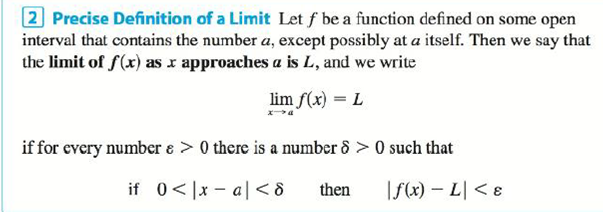Chapter 2.4, Problem 38E

Chapter
Section
Textbook Problem

If H is the Heaviside function defined in Example 2.2.6, prove, using Definition 2, that lim t → 0 H ( t ) does not exist. [Hint: Use an indirect proof as follows. Suppose that the limit is L Take ε = 1 2 in the definition of a limit and try to arrive at a contradiction.]Definition 2To determine

To prove: The limit of a function limt0H(t) does not exist.

Explanation

Definition of a limit:

Let f be a function defined on some open interval that contains the number a, except possibly at a itself. Then the limit of the f(x) as x approaches a is L, that is

limxaf(x)=L

If for every number ε>0 there is a number δ>0 such that

if 0<|xa|<δ then |f(x)L|<ε.

Proof:

Suppose that limt0H(t)=L.

By the definition of a limit,

Given ε=12 there exist δ>0 such that 0<|t|<δ implies

|H(t)L|<ε|H(t)L

Still sussing out bartleby?

Check out a sample textbook solution.

See a sample solution

The Solution to Your Study Problems

Bartleby provides explanations to thousands of textbook problems written by our experts, many with advanced degrees!

Get Started

Fill in the blanks. 4. An equation of the circle with center C(a, b) and radius r is given by __________.

Applied Calculus for the Managerial, Life, and Social Sciences: A Brief Approach

What condition on and is necessary for the standard beta pdf to be symmetric?

Probability and Statistics for Engineering and the Sciences

The general solution to dydt=ky is: a) y(t) = y(0)ekt b) y(t) = y(k)et c) y(t) = y(t)ek d) y(t) = ey(0)kt

Study Guide for Stewart's Single Variable Calculus: Early Transcendentals, 8th

Fill in the blanks. f-gx= __________

College Algebra (MindTap Course List)